# 1.本章学习总结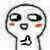## 1.1 思维导图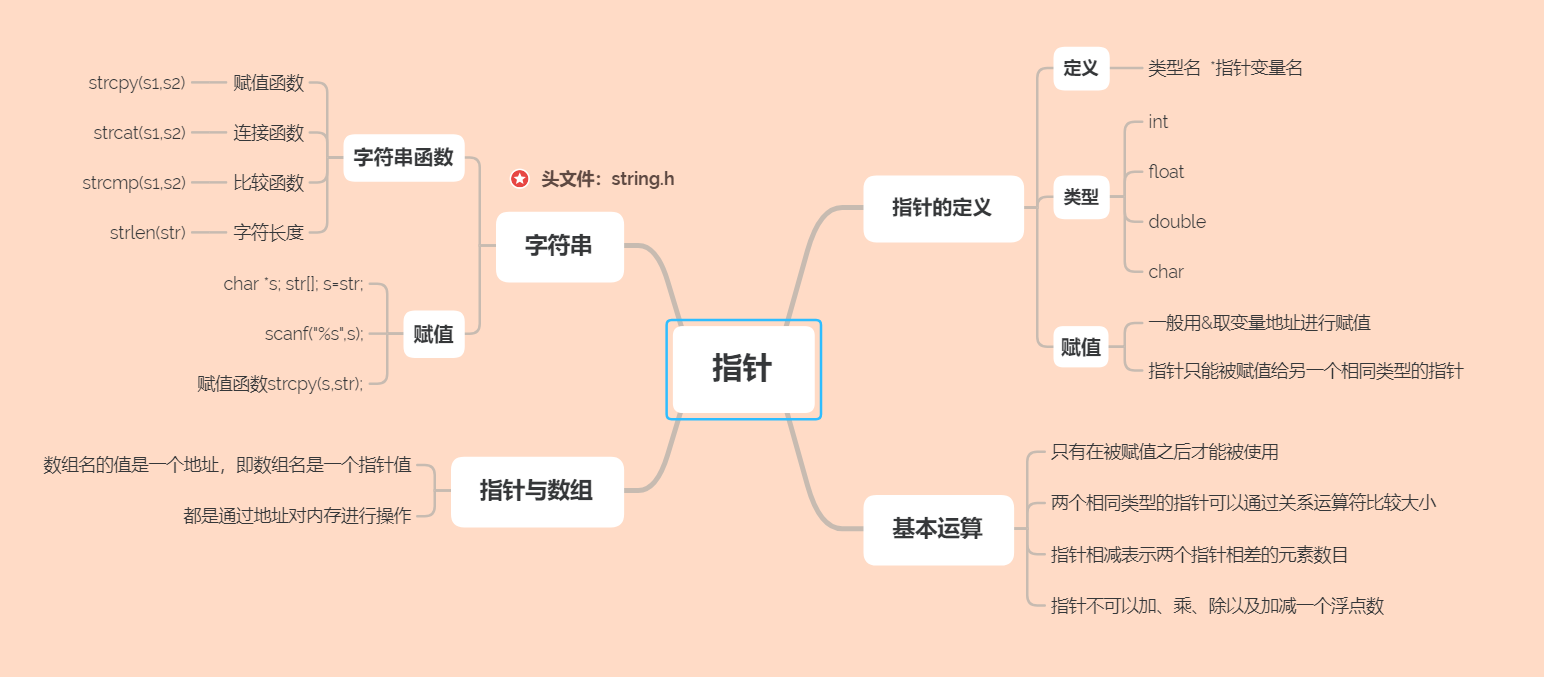## 1.2 本章学习体会及代码量学习体会

### 1.2.2 代码累计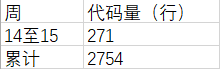# 2.PTA总分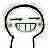## 2.1截图PTA中指针题目集的排名得分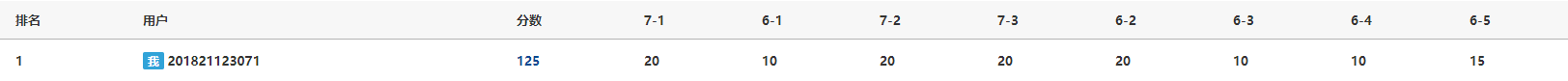125分

# 3.PTA实验作业## 3.1 PTA题目

### 3.1.1 算法分析

定义 i,统计count=0;

gets(s); //输入英语
i=strlen(s)-1;//取字符串长度
for i=strlen(s)-1 to 0   do
if s[i]==' '   then
if s[i+1]!=' '&&s[i+1]!='\0'  //出现单词
p=s+i+1;
count++;
if count>1  then
printf(" ");//控制输出最后一个单词没有空格
printf("%s",p);
end if
s[i]='\0';
end if
else if i==0&&s[i]!=' '  then//单独判断最后一个
p=s;
if count>=1 then
printf(" ");
end if
printf("%s",p);
end
end for


### 3.1.2 代码截图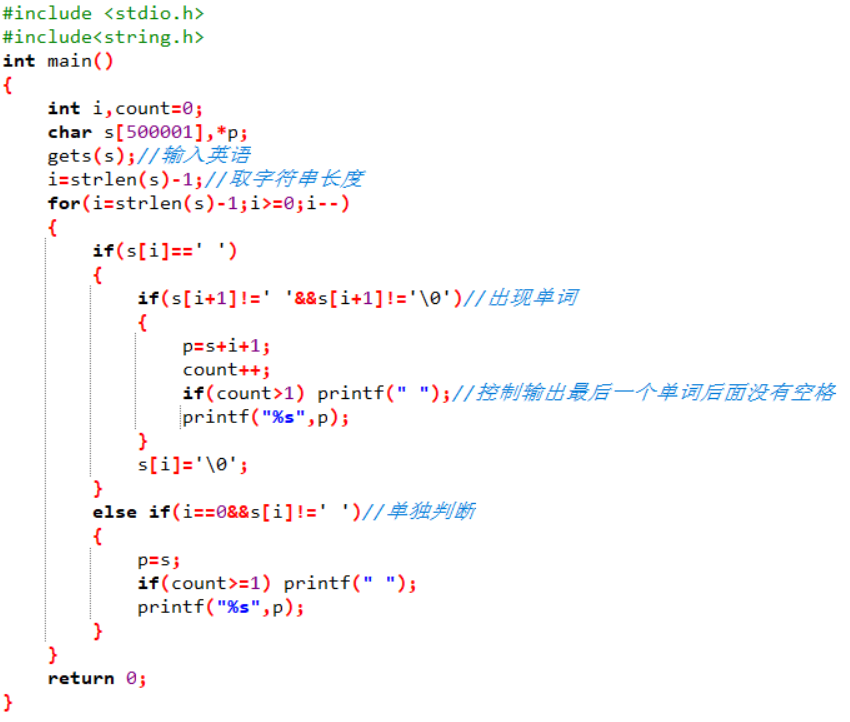### 3.1.3 PTA提交列表及说明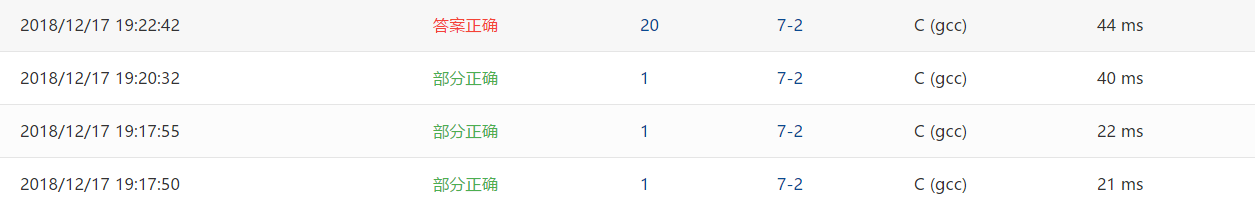-Q1:刚开始在输出方面没有什么思路
-A1:后来去请教了同学
-Q2:一开始没有发现最后单词没有空格
-A2:通过count控制

# 4.大作业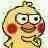## 4.1.改造函数介绍

### 4.1.1 函数

函数CreateExp(char *exp,int level)
定义整型变量 题量number,答题次数times,统计count=0;item,temp;
定义整型变量 i,j,符号sign,数字个数k,位数m,运算符种类n,a;
static char str,ch;
static int num;//定义整型数组 数字num并初始化
提示并输入题量;
for i=1 to number do
memset(exp,0,100*sizeof(char));//每次输出后将存放表达式的指针的内容清空
if level=1 then
k=2;m=9;n=4;a=1;break;
else if level=2 then
k=3;m=90;n=2;a=10;break;
else if level=2 then
k=3;m=900;n=2;a=100;break;//根据级别，赋予对应的参数
num=level;//将存放数字的数组的首位存放级别
for j=1 to k do num[j]=rand()%m+a;//生成随机数字
for j=1 to k-1 do
sign=rand()%n+1;
switch(sign)
case 1:ch[j]='+';break;
case 2:ch[j]='-';break;
case 3:ch[j]='*';break;
case 4:ch[j]='/';break; //随机生成运算符，并储存在数组中；
Select(num,ch);//判断数字是否满足条件
for j=1 to (k*4) do
if j==1 or j==5 or j==9 then
itoa(num[temp],str,10);//将数字转换为字符
temp++;
else if j==3 or (j==7 and level!=1) then *str=ch[j/3];
else if j==(k*4)-1 then *str='=';
else *str=' ';
strcat(exp,str);
memset(str,0,100*sizeof(char));//清空 *str
输出算式，并提醒用户输入答案;
询问是否中途退出;
Rate(count,times)*100);//调用函数计算正确率


void *memset(void *s, int ch, size_t n)；头文件在<string.h>中，将s中当前位置后面的n个字节 (typedef unsigned int size_t )用 ch 替换并返回 s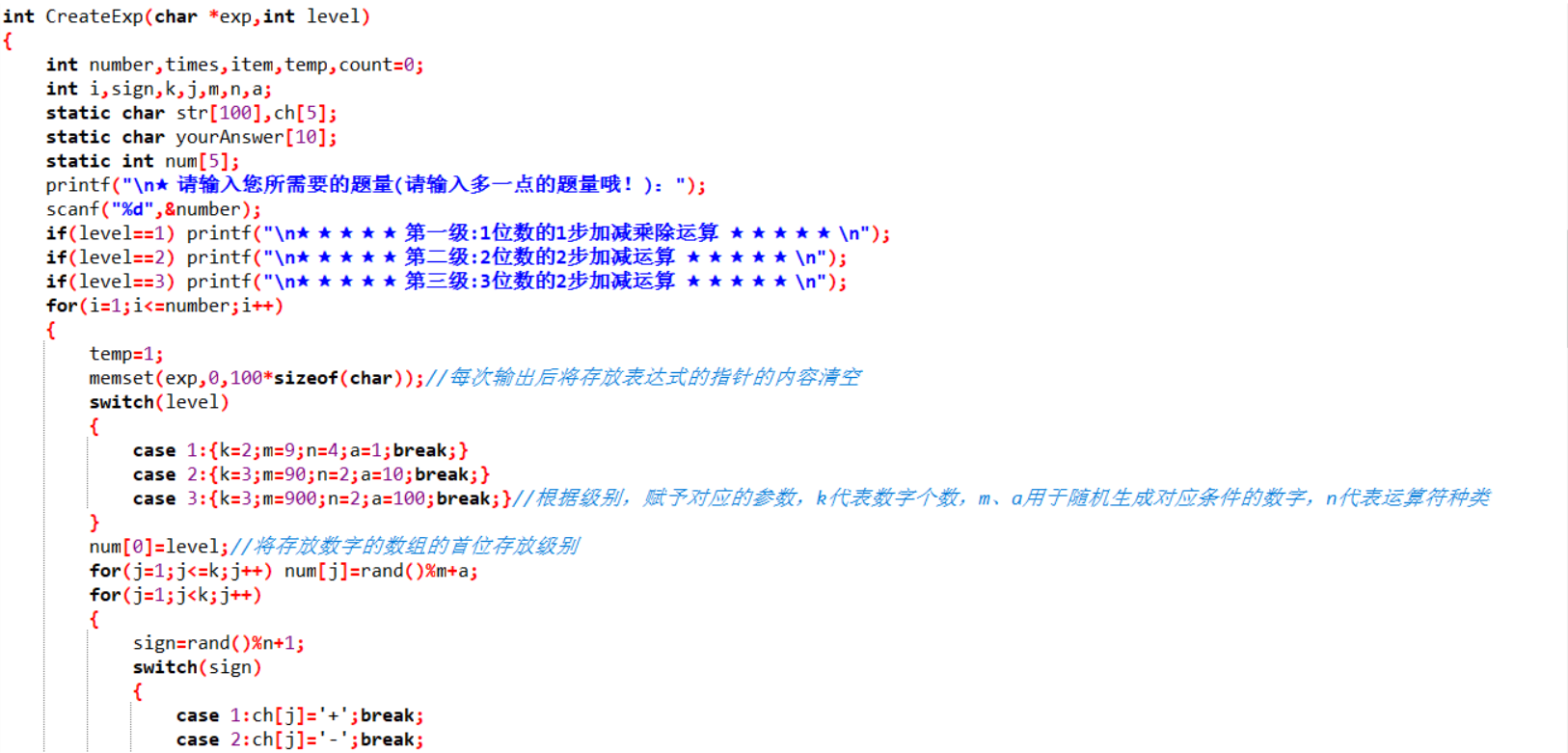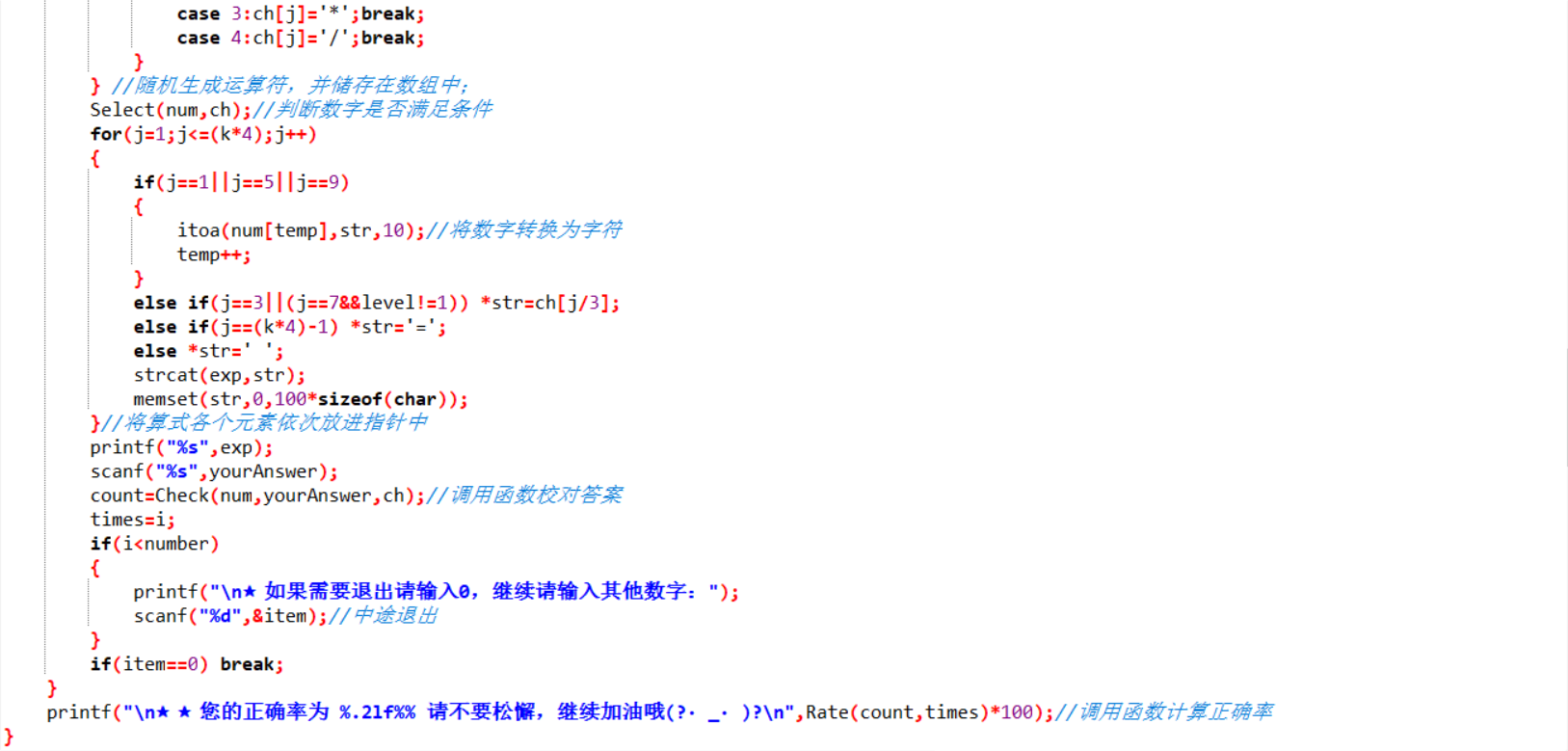### 4.1.2 函数

函数Select(int *num,char *ch)
定义整型变量 change,result;
if num<num and ch=='-'
通过变量change将两者进行交换//使1位数运算结果为正数
if ch=='/' then
while num%num!=0
重新生成两个运算数//将除法定义为整除运算
if num!=1 then 计算前两个数字运算结果
while result<num
if ch=='+' then break;
switch(num)
case 2: num=rand()%90+10;break;
case 3: num=rand()%900+100;break;//	使2位数和3位数运算结果为正数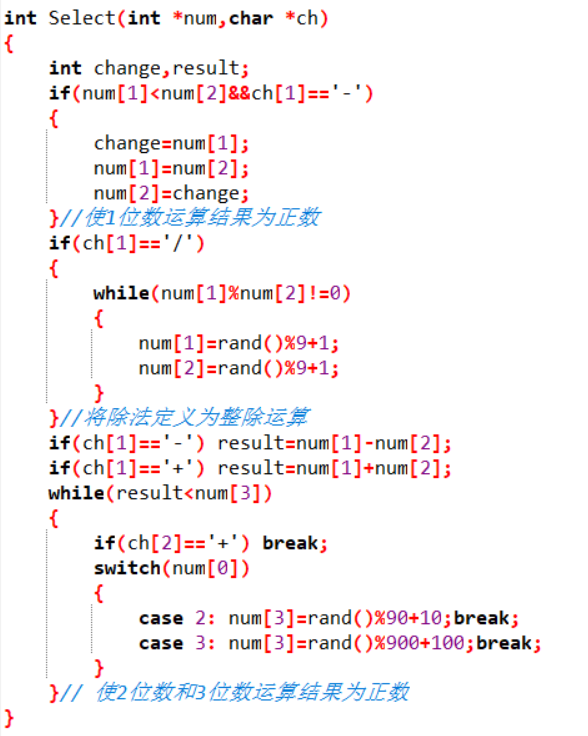### 4.1.3 函数

函数Check(int *num,char *yourAnswer,char *ch,int count)//计算正确率
static char str;//定义字符串str并初始化
switch(ch)
根据符号进行计算;
switch(ch)
根据符号进行计算;
default:  break;//若只有1步计算则break
输出答案正确
count++;
else 提示答案错误并输出正确答案
return count;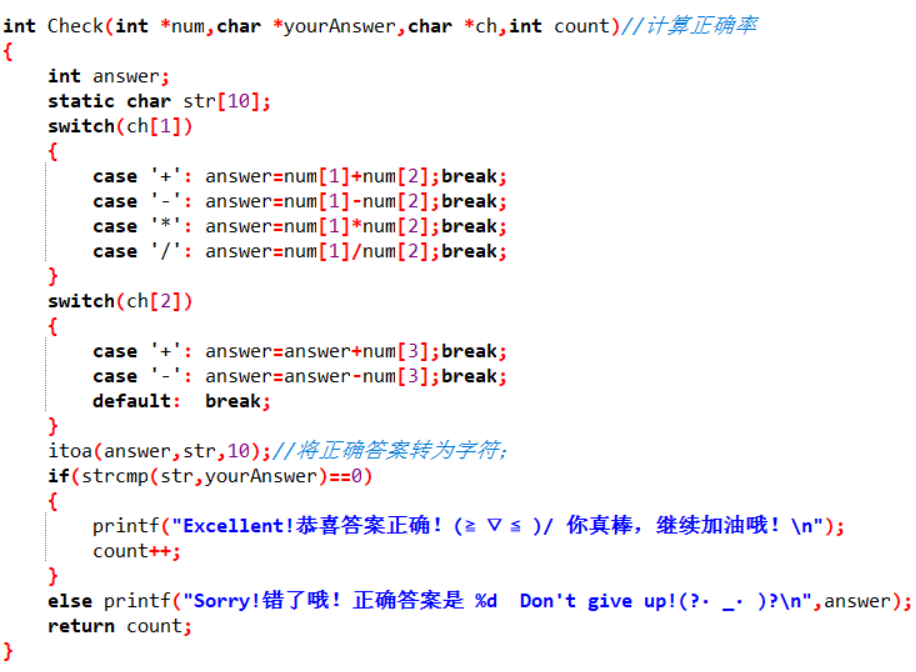## 4.2 与原有函数代码比较

### 改造前函数1、2和改造后的函数1、2

• 改造前的函数1、2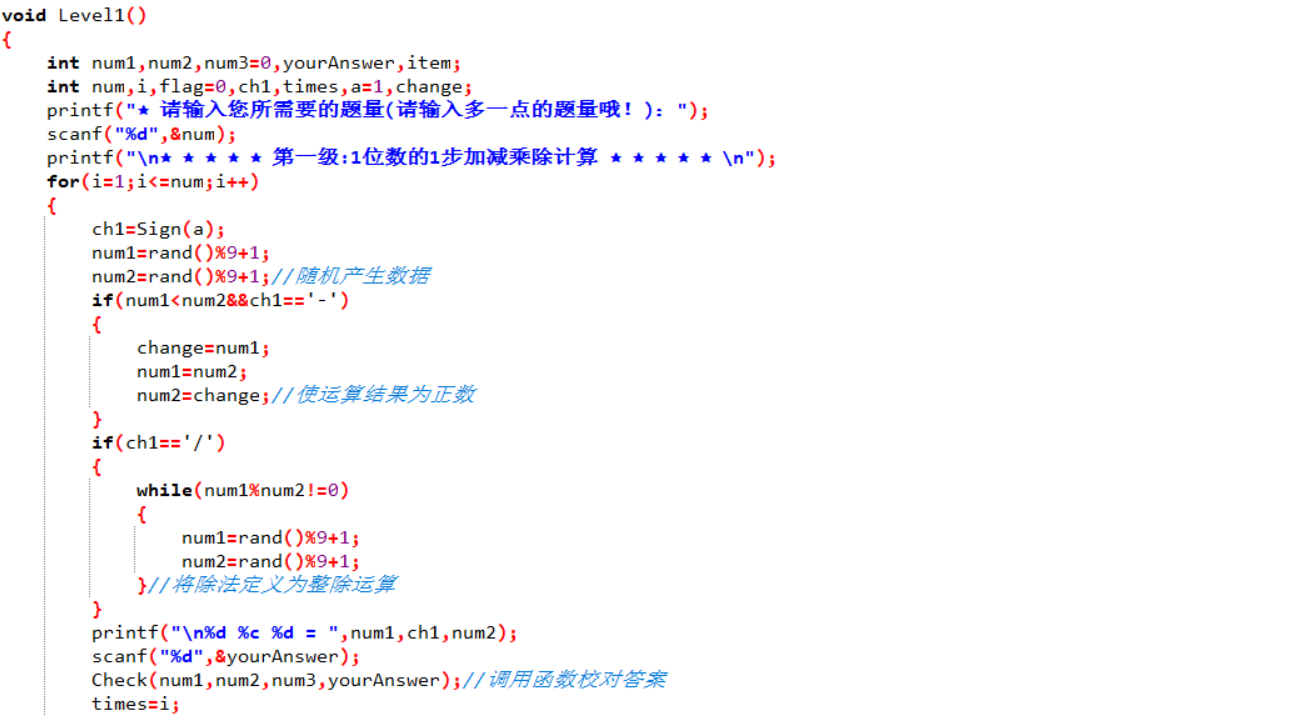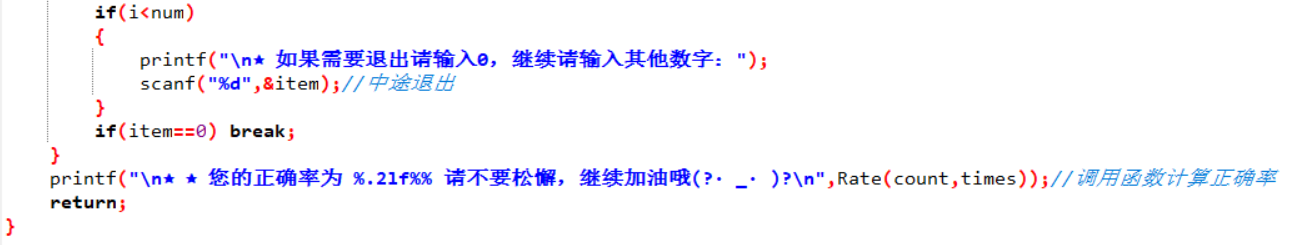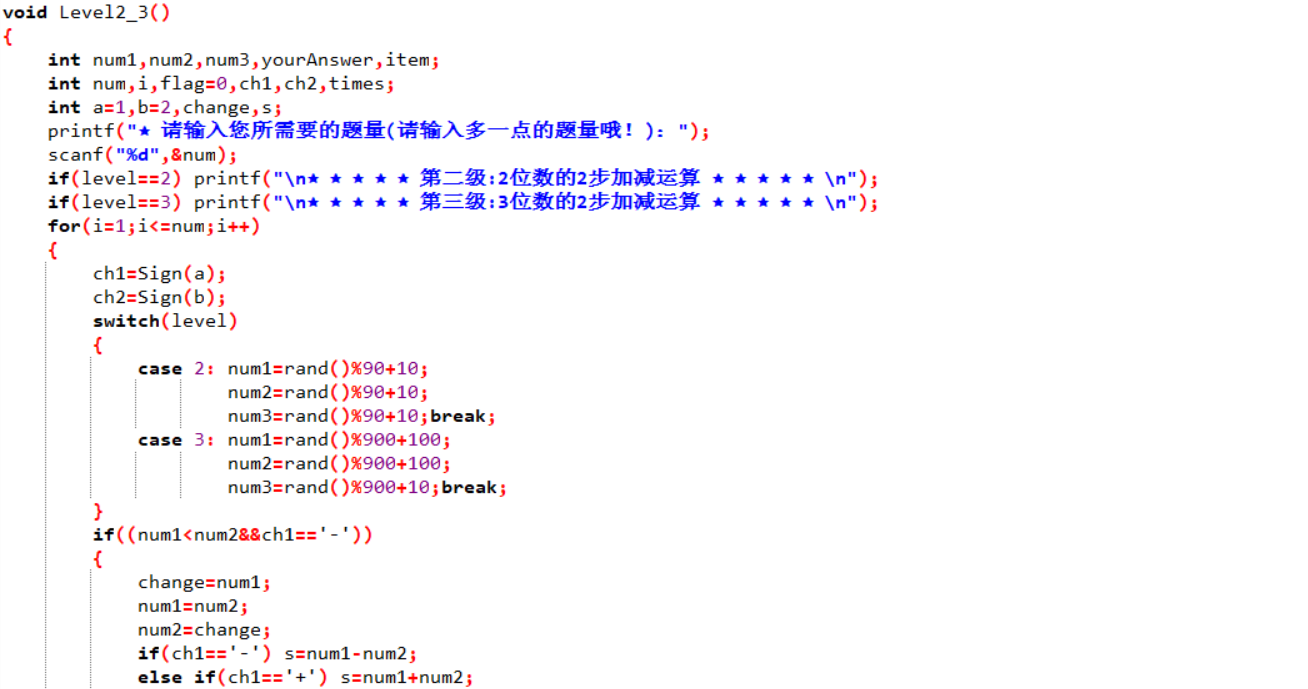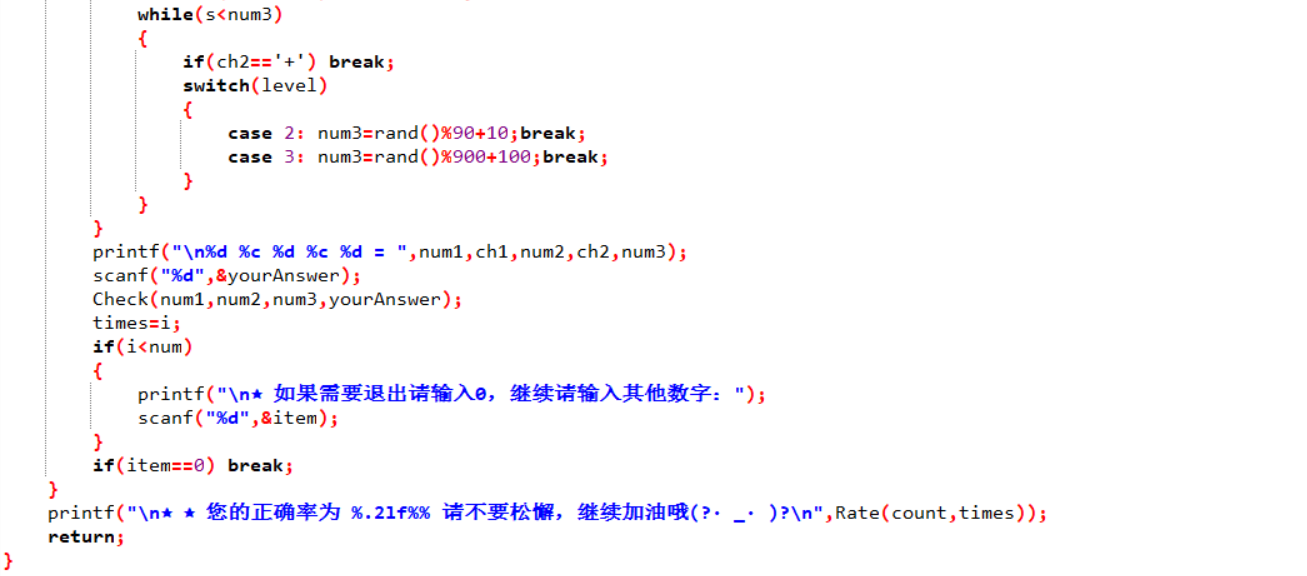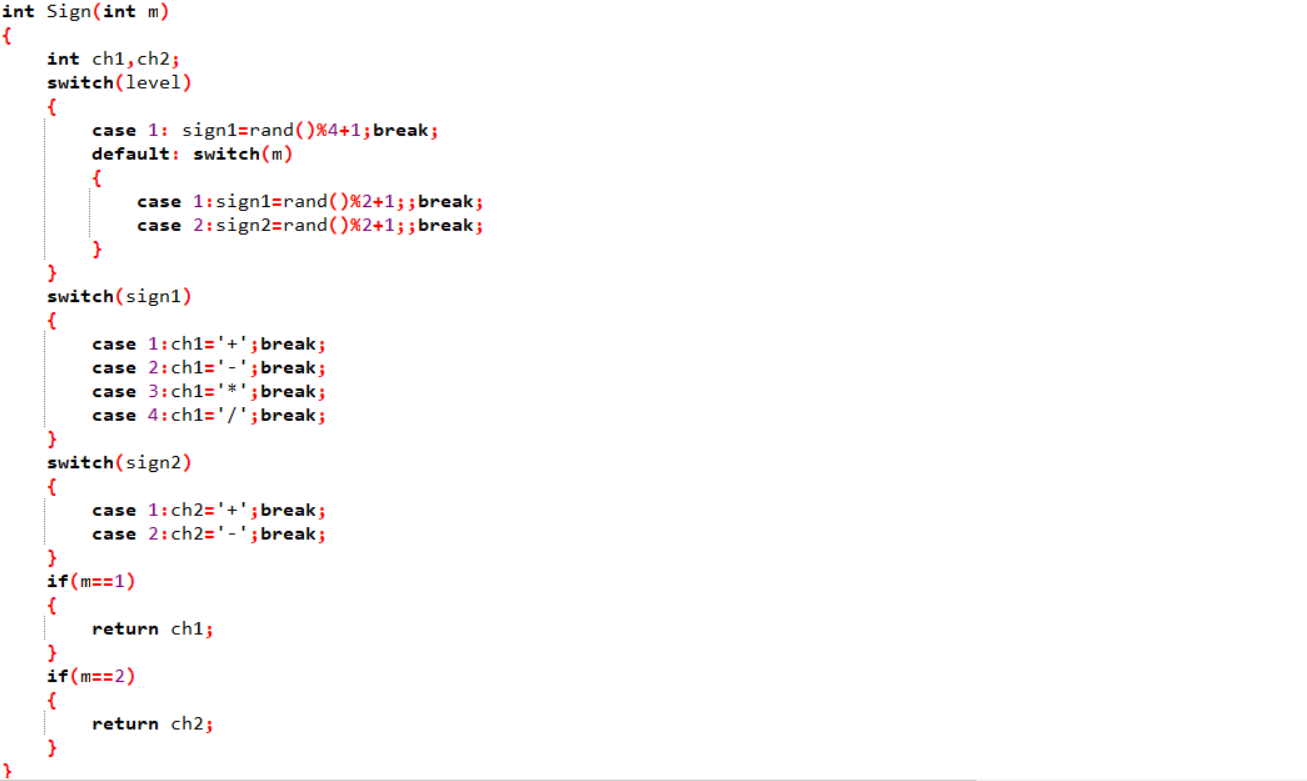• 改造后的函数1、21.函数封装的不同，改造前将级别1、级别2&3和符号生成的函数是依次分开，而改造后的是封装成生成运算式和判断运算式是否合理两个函数；
2.在生成运算式这一块，是定义多个变量，每个变量对应一个值，而改造后是存放在数组中

1.改造前将级别1、级别2&3是分开的，许多语句是重复的，不利于阅读，改造后将一些重复的语句删除；
2.通过指针，取消了全局变量

### 改造前函数3和改造后的函数3

• 改造前的函数3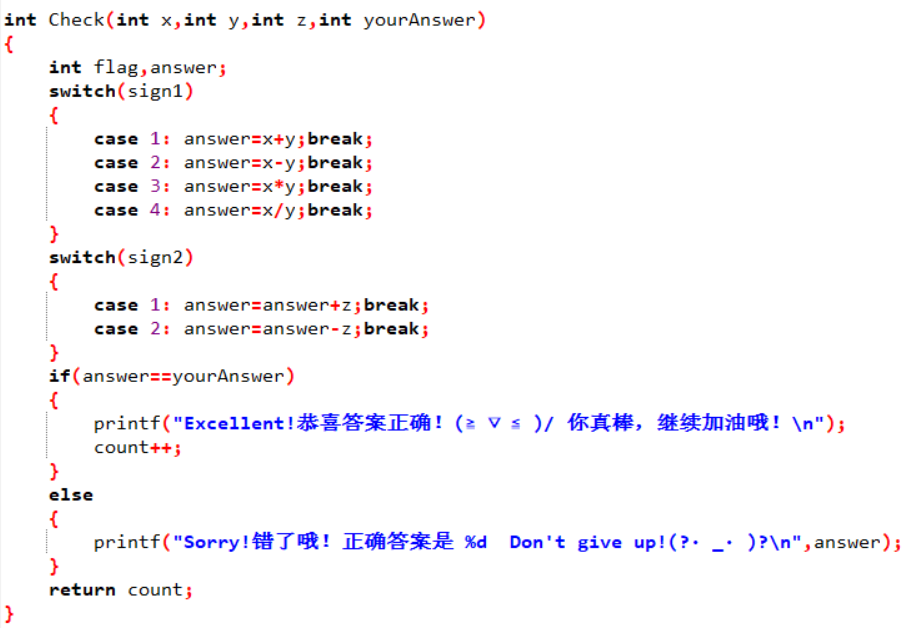• 改造后的函数31.改造后传递的输入答案为字符串；
2.改造后通过传递数组计算正确答案

1.改造后传递的输入答案为字符串，完善了之前输入字符串时程序会直接结束的bug

## 4.3 改进大作业总结

posted @ 2018-12-17 20:18  _TY  阅读(278)  评论(2编辑  收藏  举报